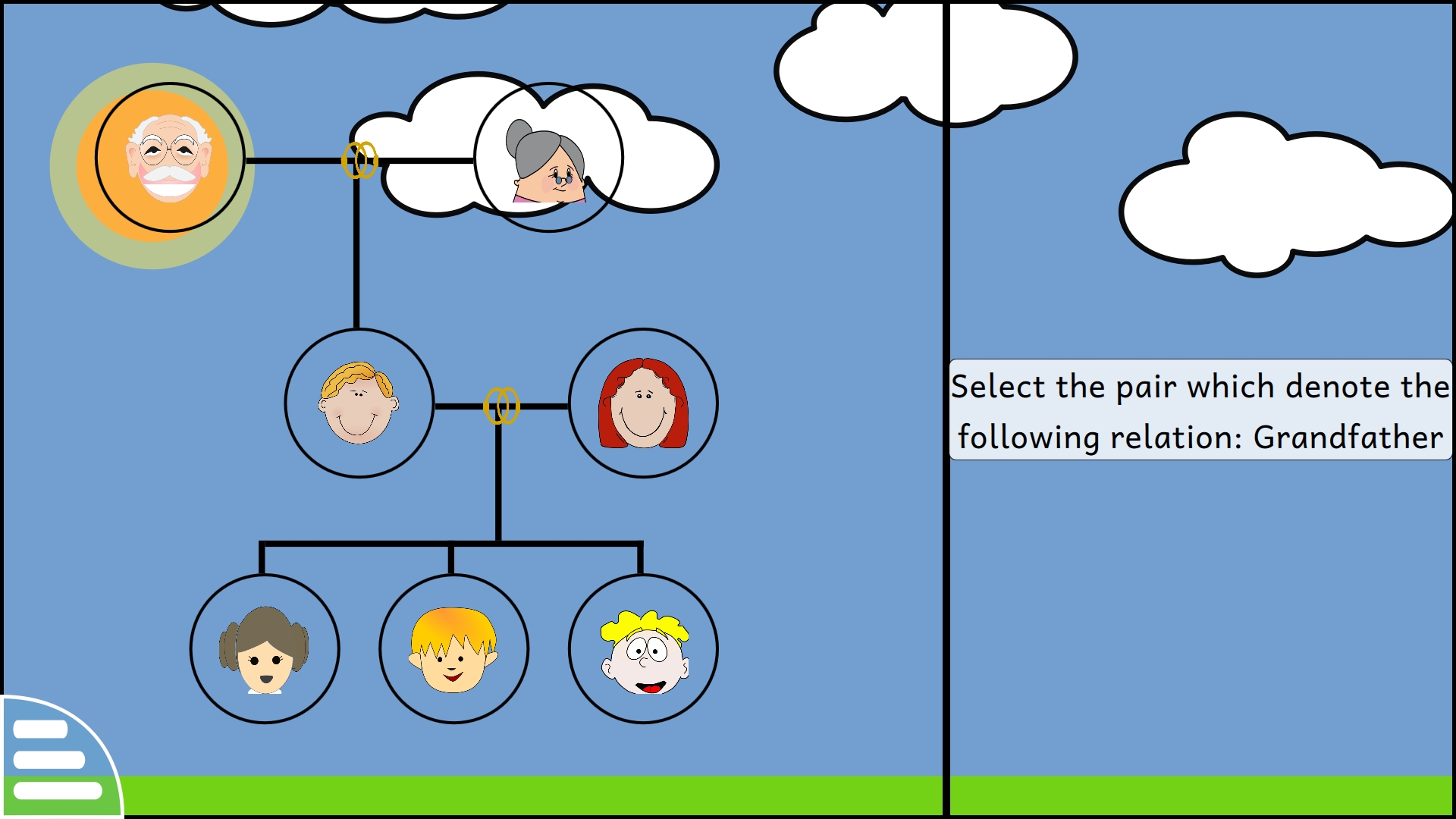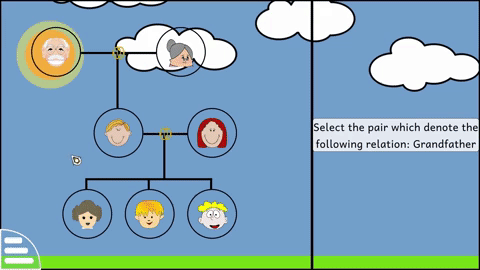# Family - Find relativesFamily_find_relatives is an extension of the Family activity which is aimed at providing a bit more challenge for someone who has already finished the family activity. The goal of this activity is: given a relation, the user will have to select a pair of node that correctly represents the given relation.

### Apparent Solution

The implementation of the activity initially seems to be a straightforward one. We will just store the pair of nodes which are selected, and if one of them is of the type “active” and the other is of the type “activeTo”, then the answer will be marked as correct, else incorrect.

But on closer look, it can be seen that this approach is flawed. It may be possible that there are more than one existing pairs which satisfy the given relation and whose `state` may be “deactive”.There are three pairs which can satisfy the given relation

### Implementation

#### Pair matching

To overcome the above problem, we add another property for every node in the current level.

``````readonly property int pair_1: -1
``````

We add the above properties in the `Dataset.qml` file, to keep a track of which nodes can take part in the pairing process, using the following logic:

• All nodes in a given level has a `nodeWeight` value, which can be either `no_pair`, `pair_1` or `pair_2`
• For a selected pair of nodes to be a correct one, one of the node value should be of the type `pair_1` and the other should be of the type `pair_2`. Any other combination is marked as a “Wrong Answer”#### Randomising levels

After testing the activity several times, it was noticed that the activity gets predictable once the original family activity is already played by the user. To overcome this, we decided to shuffle the levels everytime the activity is loaded. This is implemented by:

``````function shuffle() {
if (items.mode == "normal") {
// not required for normal mode
return
}

for (var i = 0;i < numberOfLevel;i++) {
shuffledLevelIndex[i] = i
}

var currentIndex = shuffledLevelIndex.length, tmp, randomIndex

while (currentIndex != 0) {
randomIndex = Math.floor(Math.random() * currentIndex)
currentIndex -= 1

tmp = shuffledLevelIndex[currentIndex]
shuffledLevelIndex[currentIndex] = shuffledLevelIndex[randomIndex]
shuffledLevelIndex[randomIndex] = tmp
}
}
``````

`shuffledIndex[]` contains the indices of the levels and when we have to load data from a dataset, we do it by:

``````// get the index of the level to load
levelToLoad = items.mode == "normal" ? currentLevel : shuffledLevelIndex[currentLevel]

// load the appropriate dataset by the index